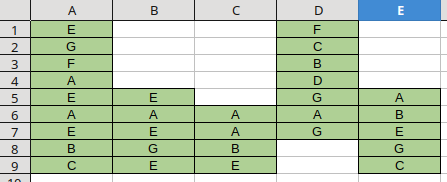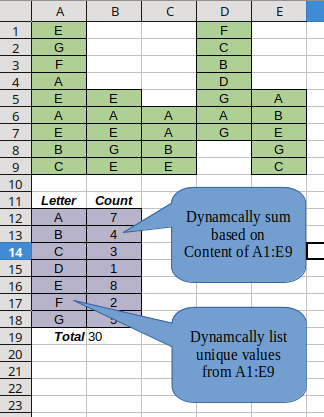# "How to:" Scan array and produce summary

I’m sure I’m missing the obvious if so, please accept my apology.

In calc, I have a page that looks like this:(Yes, this is the simplified version.)

I’d like to be able to automate the process of producing a summary of these cells.

I’m thinking of trying to produce something like:(Constraint: Contents of cells not predefined but X/Y geography is.)

I started searching here and couldn’t sort out what keywords I should be searching for.

I’m sure I’m not the only one that’s headed down this road so I’m asking for pointers of what I should be looking for (keywords) or advice of just where to start.

However, if anyone thinks it’ll make a good addition to some FAQ some place, by all means, dive in.Thanks in advance for any pointers.

Probably, this task can be solved using formulas and a pivot table. But I decided that writing a macro would be faster:

``````Function showStatistic(aData As Variant) As Variant
Dim i As Long, j As Long
Dim aTemp As Variant
Dim aResult As Variant
aTemp = Array()
Next j
Next i
aResult = DimArray(UBound(aTemp),1)
For i = LBound(aTemp) To UBound(aTemp)
aResult(i,0) = aTemp(i)(0)
aResult(i,1) = aTemp(i)(1)
Next i
showStatistic = aResult
End Function

Sub countUnique(key As Variant, aData As Variant)
Dim l&, r&, m&, N&, i&
N = r
While (l < r)
m = l + Int((r - l) / 2)
l = m + 1
Else
r = m
End If
Wend
If r = N Then
Else
For i = N - 1 To r Step -1
Next i
End If
End Sub
``````

Just write in a cell something like `=SHOWSTATISTIC(A1:K23)` and press Ctrl+Shift+Enter

Holy crap1 That’s amazing!
Thank you!

I’ll give it a shot right now.

Hey, put your name on it!Works great! Thank you!